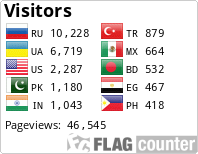Hi.
my own new invention. dividing on zero. trigonometry on logic values. 3d bit.
.
I invented 3D logic for computing systems making bit to be 3D which means more stability.
For that I introduced arithmetical operations of subtraction and division to set of logical operations.
And new logical value
Empty.
New logical arithmetic looks that way:
True = True / True
False = False / True
Empty = True / False
Empty = False / False
Empty = True / Empty
Empty = False / Empty
Empty = Empty / Empty
False = True – True
True = True – False
- True
= False – True
False = False – False
Empty = True – Empty
Empty = Empty – True
Empty = False – Empty
Empty = Empty – False
Empty = Empty – Empty
2True = True + True
True = True + False
Empty = True + Empty
False = False + False
Empty = False + Empty
Empty = Empty + Empty
power( True) = True * True
False = True * False
False = False * False
Empty = True * Empty
Empty = False * Empty
Empty = Empty * Empty
Not Empty = Empty
Not True = False
Not False = True
True Xor True = False
False Xor True = True
Empty Xor Empty = False
False Xor Empty = Empty
True Xor Empty = Empty
-(True) = -True
-(False) = False
-Empty =
Empty
-True Xor True = False
-True Xor False = -True

Empty is produced almost by all operations with participation of Empty, and also by division on False and 0, and by all errors in type cast, and all unresulting functions including divisions of matrices and trees.
Introducing of operation of division to set of logic operations is making possible the application of trigonometric functions to logical values which is much easing the search of True and making possible the introducing of trigonometrical functions for Subject, Object, and Predicate and not only that.
I have many ideas for new programming languages. I develop model in which used C-like language Cross, LISP-like language Word, and language Think emulating work of mind and retrieving elements of system including hierarchical Database.

All languages of system work on one virtual machine which empowers instruments of artificial intelligence.
With all due respects
Sergey Streltsov